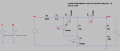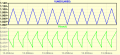# Is it possible to calculate capacitor voltage in this case?

#### anhnha

Joined Apr 19, 2012
901
Regardless of the uselessness of this boost converter as it only works with 50% duty cycle in order to satisfy charge balance condition for capacitor C1, is the voltage across C1 undefined in this case?Simulation shows that voltage across C1 is around V1 = 1V at steady state.Is it possible to calculate that voltage? I don't see any constraint for V1 except for charge balance condition with 50% duty cycle.
It seems like any value of V1 is possible (assume that open loop no feedback regulation for Vout).

#### Attachments

• 2.7 KB Views: 10

#### LowQCab

Joined Nov 6, 2012
1,925
I would at least double the Input-Voltage because of the Inductor being in the Circuit.
The Input Resistor "may" make this over-kill.
.
.
.

#### MrAl

Joined Jun 17, 2014
8,868
Regardless of the uselessness of this boost converter as it only works with 50% duty cycle in order to satisfy charge balance condition for capacitor C1, is the voltage across C1 undefined in this case?

View attachment 253956

Simulation shows that voltage across C1 is around V1 = 1V at steady state.

View attachment 253957

Is it possible to calculate that voltage? I don't see any constraint for V1 except for charge balance condition with 50% duty cycle.
It seems like any value of V1 is possible (assume that open loop no feedback regulation for Vout).
Why wouldnt it be possible to calculate that voltage (C1)?
That is, what would make you think you cant?

#### anhnha

Joined Apr 19, 2012
901
Why wouldnt it be possible to calculate that voltage (C1)?
That is, what would make you think you cant?
Because I don't see any constraint for VC1 except 50% duty cycle to ensure the charge balance for the capacitor.
Also the output is always 2Vin with any value of VC1.

#### MrAl

Joined Jun 17, 2014
8,868
Because I don't see any constraint for VC1 except 50% duty cycle to ensure the charge balance for the capacitor.
Also the output is always 2Vin with any value of VC1.
Ok well let me see if i can understand this from your point of view so that i can help the most here.

Why are you looking for a constraint for the capacitor voltage (C1) ?
Is it that you are looking for a shortcut or trick to understand this more quickly than a full blown analysis? I could understand that because we often see shortcuts appear for various circuits, but i believe they originate from a full analysis. So you would do an analysis with different starting conditions, then come up with a conjecture of what might be happening from a simpler standpoint, then try to prove that, then repeat if the proof doesnt pan out. If you can do a formal symbolic analysis, then the formula will spill out from the resulting equations although this gets more and more difficult as you go up in order (2nd order circuits are quite simple, 3rd order a lot harder, 4th order very complicated, 5th order probably not worth doing. These get simpler if you have fixed component values though).

For example, for a buck circuit we know now that the output is (ideally) Vout=Vin*D. But where did that come from? It came from doing a full analysis and then analyzing the data to come up with that formula, then proving it with various perturbations. For example, if you double the input voltage you may get a lower output but it may be half of the input and that holds for many input values as long as the duty cycle is 50 percent. Then if you change the duty cycles to 25 percent you see 1/4 of the output now and that holds for all inputs as well. Eventually you build a case for Vout=Vin*D and that becomes similar to a control law that holds in the ideal case. You can then go on to form ideas about what happens when the catch diode is non ideal with some voltage drop like 0.5 volts, then work that into the formula. So you end up with a formula for a buck circuit without doing a full analysis with differential equations or whatever.

But to start you should probably find out how to analyze these kinds of circuits in full. If not, then you will have to make changes in the simulation and try to come up with some idea of what is happening to that voltage. I think you are doing it this way already. All you have to do then is try more values of input for example and maybe duty cycle. Make a chart of what the capacitor voltage or average capacitor voltage is (or whatever quantity you are shooting for) and then try to come up with a simple formula that is able to either get this value exactly or to some approximation like 1 percent, although 1/2 percent or better would be the usual goal.

See what you can do now and let me know what you find.

#### anhnha

Joined Apr 19, 2012
901
Why are you looking for a constraint for the capacitor voltage (C1) ?
I don't know if there exists another constraint of VC1. I'm trying to find out how to calculate VC1 in that case.
I did a an analysis to find the voltage conversion ratio.
Voltage - second balance condition for the inductor:
D*T (Vin - VC1) + (1-D)T*(Vin + VC1 - Vout) = 0
from this I solved for Vout = (Vin + (1-2D)VC1)/(1-D). However, D should be 0.5 to satisfy charge balance for C1 in stead state.
So Vout = 2Vin for every VC1.
I don't see any constraint for VC1 in order to calculate its value.

•MICROWHIZZ